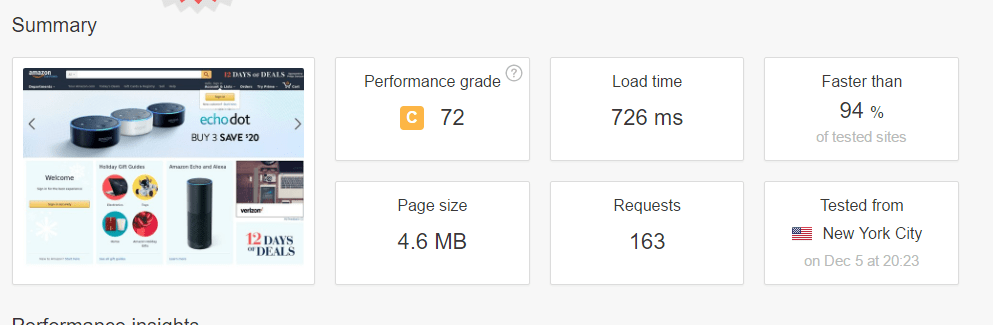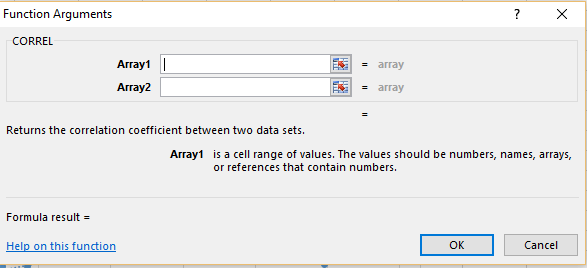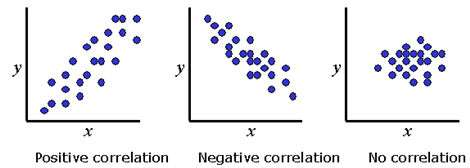# What does Google Page Speed Score Measure?

Google Page Speed Insight Score is often used to grade the speed of a website. However, the same question always arises; is this an accurate measurement. The answer may lie in Google’s own definition of their test. Google defines the Page Speed Insights as a correlation of network-independent variables that can have an affect on page performance such as the server configuration, the HTML structure of a page, and its use of external resources such as images, JavaScript, and CSS.

So what does this mean? This means that the test is a measure of coding best practices and does not take in consideration the technology utilized that also significantly can boost page load time. Tech like CDN and asynchronously loading of critical UX elements is not considered. So in many cases, a fully rendered page can load in seconds leaving social media or non essential elements to load later.

If you work in the industry of marketing, eCommerce, or web design, you know the importance of having a fast website. You are constantly chasing that page load speed metric. However, speed performance test will not always equate to page load speed. Take a look at the Pingdom test load time is 726ms for Amazon.com. However the performance score is 72.To add insult to industry. Amaazon receives an even less effective score when using the Google Page Speed Insights Test.## What is the connection between page speed and Google Score?

So the goal was to measure the correlation between the variables, load time and the Google Page Speed Insight Score. First, method used was the correlation formula to measure the correlation of the 50 websites. The websites were sourced from Wikipedia and Quantcast. The tool used to calculate the page speed was Pingdom Tools.

This may look complicated. However, it’s not. There are a lot of software packages that will allow you to quickly calculate the correlation coefficient. For example in Excel you can calculate this by imputing the CORREL formula. You can learn more about Correlation in Excel if this is your primary stats tool.## Using Linear Regression

In order to model the relationship between the two variables, we can use linear regression. We can use a software package in Excel to perform this analysis. However, to perform this, you need to add analysis pack to Excel. Once added, this can be found in the Data tab on the Excel ribbon.How to we interpret this?

There is a weak relationship between to the variables in terms of correlation. The multiple R is equivalent to correlation. A correlation of 1 or -1 would indicate a strong relationship between load speed and the Google Page Speed Score. However the correlation here is only 0.32.

We can get the r squared which indicates the percent in which the model explains the variability in the predicted trend line and actual scores. This ranges from 0 to 100%. So, there is only 10.6% of the variation in one variable may be explained by the other. In other words, there is a very weak relationship between the Google page speed test and load speed.Conclusion: There is a weak correlation between Google Page Speed Score and actual load speed. These two variable are not perfectly aligned. However, this does not mean that there is not real value in the suggestions given by Google. These are best practices that should in theory improve performance.

Adwords Quality Score and Weighted Quality Score Correlation

• in
• |
• October 3, 2016#### Gaelim Holland

Subscribe
Notify of